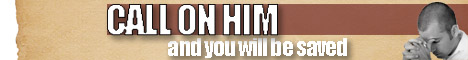Note:  Do not rely on this information. It is very old.

# Modulus

Modulus of a material is a measure of its elasticity (q.v.) when its length, volume, or shape is altered, the respective moduli being those of length, bulk, or rigidity. The modulus is the ratio of the stress to which the material is subjected, to the strain, or proportionate deformation produced. If 10,000 lbs. per square inch will extend a bar 7 inches long to the extent of 1/100th of an inch, the stress is 10,000, the strain 1/700, and the modulus of elasticity of length 10,000 divided by 1/700 =7,000,000 lbs. per square inch. [Logarithms.]